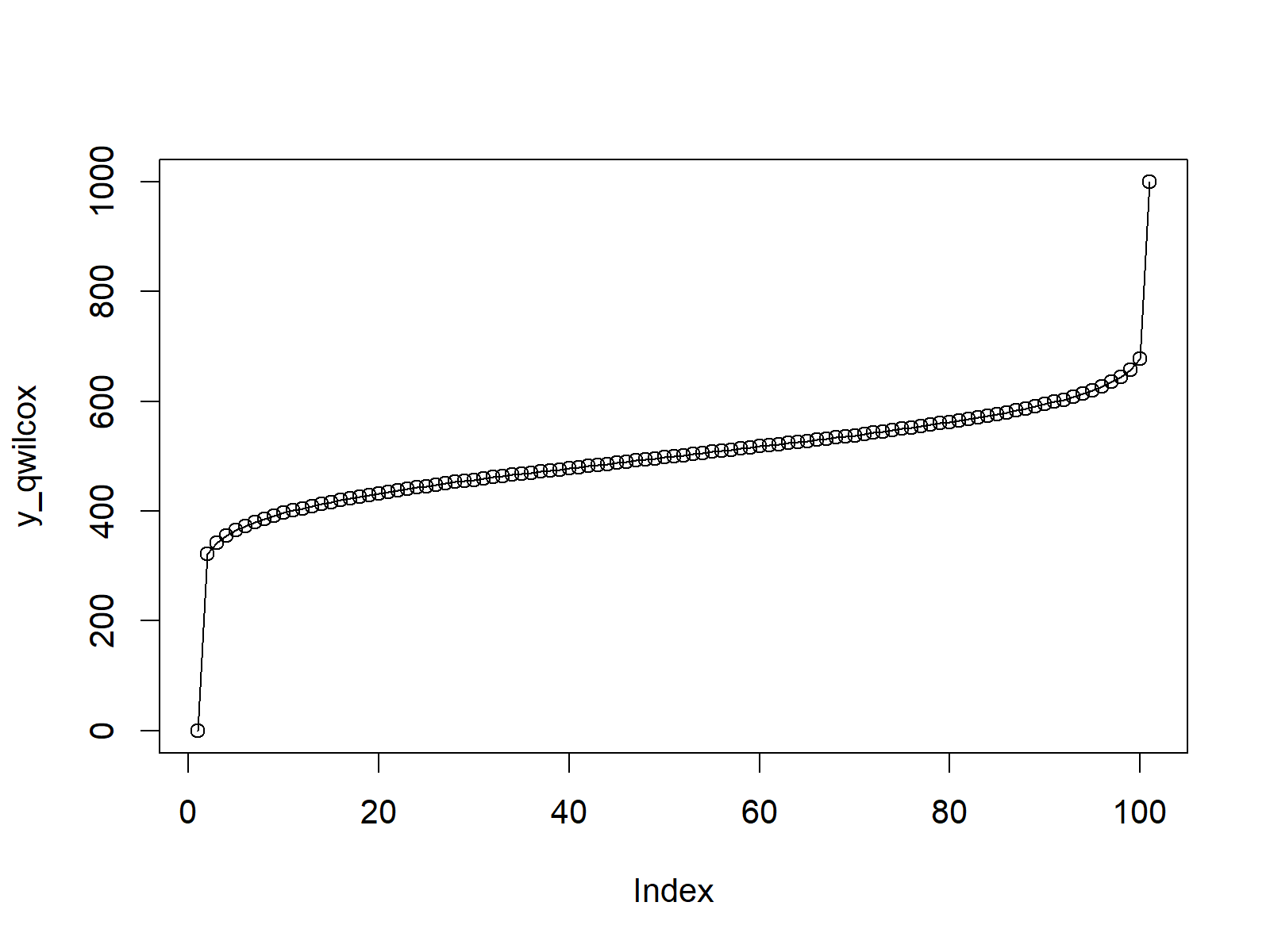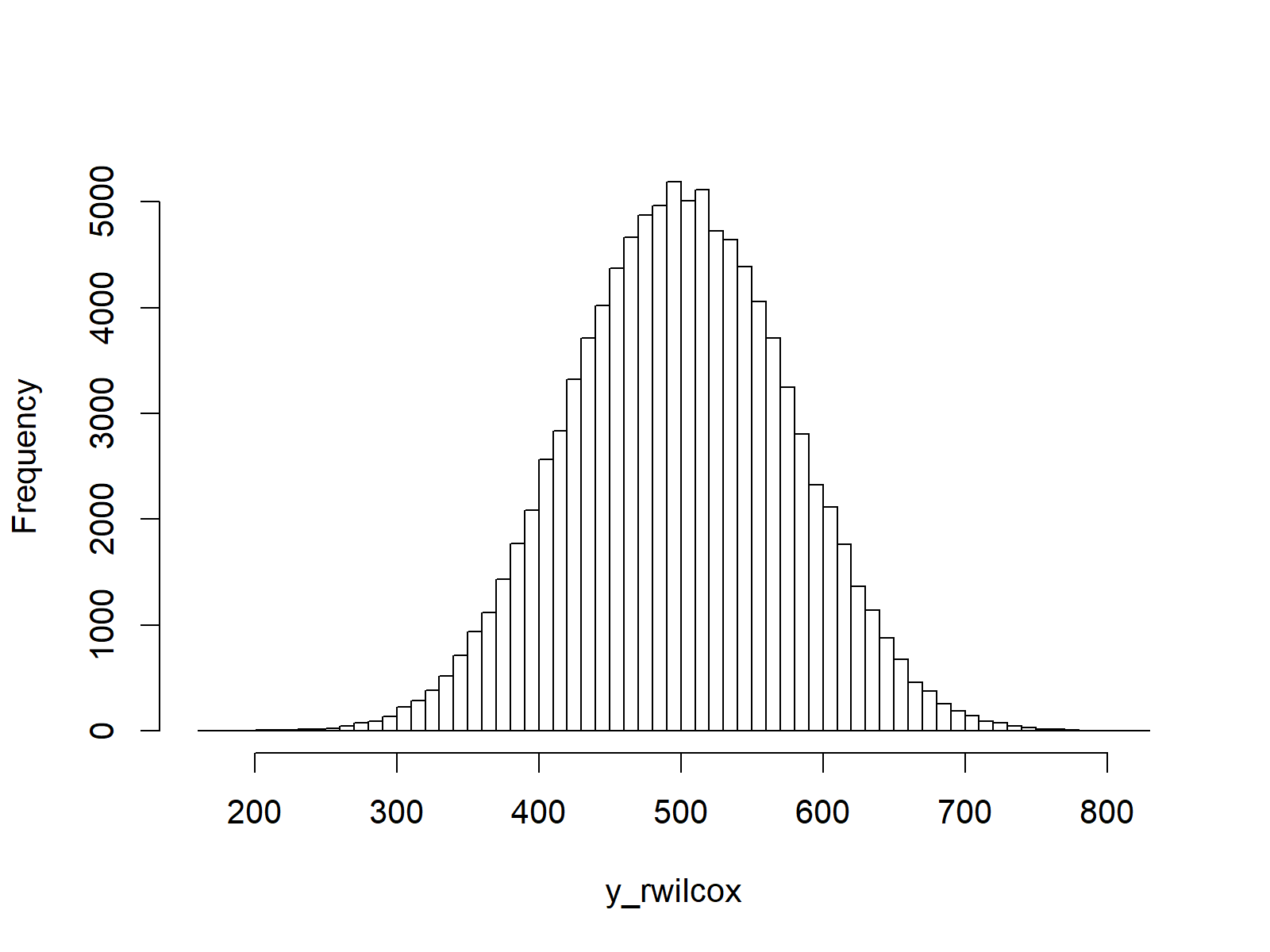# Wilcoxonank Sum Statistic Distribution in R (4 Examples) | dwilcox, pwilcox, qwilcox & rwilcox Functions

This article illustrates how to apply the Wilcoxonank Sum Statistic functions in the R programming language.

The table of content is structured as follows:

Let’s dive into it.

## Example 1: Wilcoxonank Sum Statistic Probability Density Function (dwilcox Function)

The following R code shows how to draw a graph illustrating the probability density function (PDF) of the Wilcoxonank Sum Statistic:

```x_dwilcox <- seq(0, 100, by = 1)                       # Specify x-values for dwilcox function
y_dwilcox <- dwilcox(x_dwilcox, m = 50, n = 20)        # Apply dwilcox function
plot(y_dwilcox, type = "o")                            # Plot dwilcox values```Figure 1: PDF of Wilcoxonank Sum Statistic.

## Example 2: Wilcoxonank Sum Statistic Cumulative Distribution Function (pwilcox Function)

This example explains how to draw a graphic of the cumulative distribution function (CDF) of the Wilcoxonank Sum Statistic:

```x_pwilcox <- seq(0, 100, by = 1)                       # Specify x-values for pwilcox function
y_pwilcox <- pwilcox(x_pwilcox, m = 50, n = 20)        # Apply pwilcox function
plot(y_pwilcox, type = "o")                            # Plot pwilcox values```Figure 2: CDF of Wilcoxonank Sum Statistic.

## Example 3: Wilcoxonank Sum Statistic Quantile Function (qwilcox Function)

This example shows how to create a graphic of the quantile function of the Wilcoxonank Sum Statistic:

```x_qwilcox <- seq(0, 1, by = 0.01)                      # Specify x-values for qwilcox function
y_qwilcox <- qwilcox(x_qwilcox, m = 50, n = 20)        # Apply qwilcox function
plot(y_qwilcox, type = "o")                            # Plot qwilcox values```Figure 3: Quantile Function of Wilcoxonank Sum Statistic.

## Example 4: Generating Random Numbers (rwilcox Function)

The last example illustrates how to generate random numbers according to the Wilcoxonank Sum Statistic:

```set.seed(98989)                                        # Set seed for reproducibility
N <- 100000                                            # Specify sample size
y_rwilcox <- rwilcox(N, m = 50, n = 20)                # Draw N random values
y_rwilcox                                              # Print values to RStudio console
hist(y_rwilcox,                                        # Plot of randomly drawn density
breaks = 50,
main = "")```Figure 4: Random Number According to Wilcoxonank Sum Statistic.

## Video & Further Resources

I have recently released a video instruction on my YouTube channel, which shows the R codes of this tutorial. You can find the video below:

You might also have a look at the other tutorials on distributions and the simulation of random numbers in R:

Additionally, you may want to read the related tutorials of my website:

This article explained how to use the dwilcox, pwilcox, qwilcox, and rwilcox functions in the R programming language. Don’t hesitate to tell me about it in the comments, in case you have further questions.

Subscribe to the Statistics Globe Newsletter